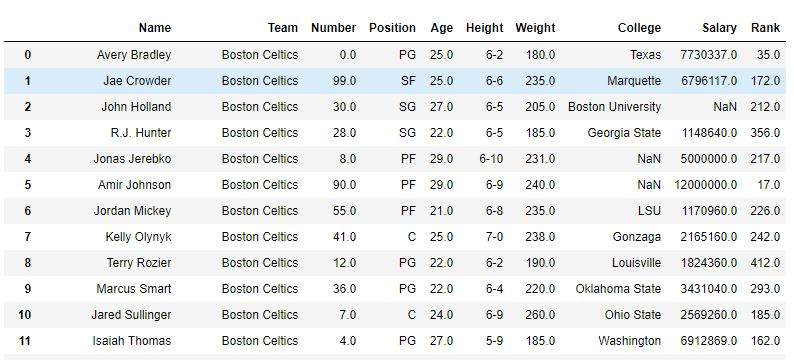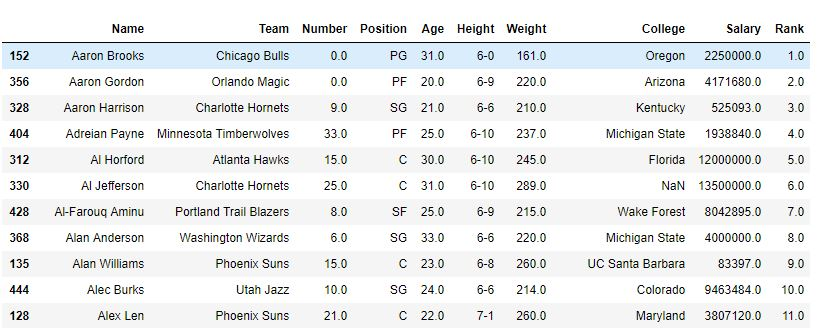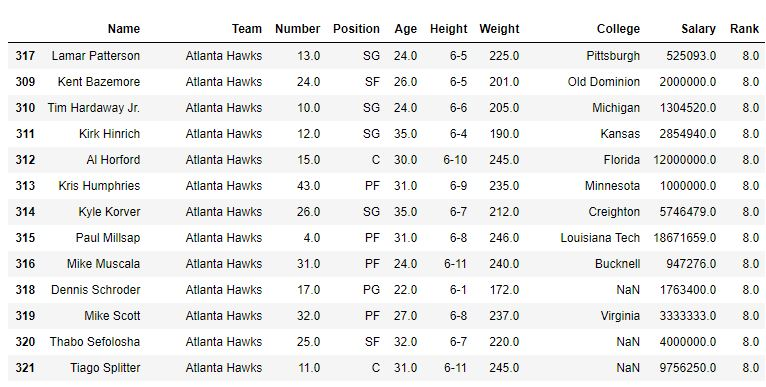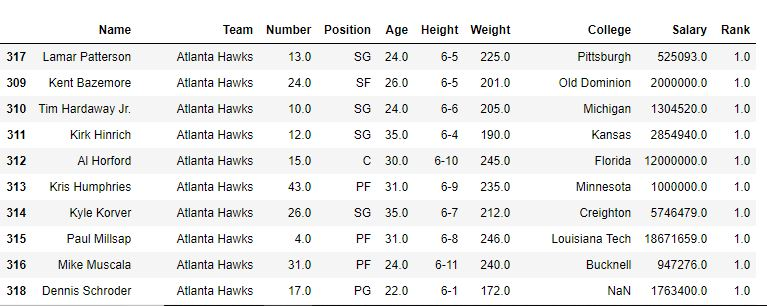# Python | Pandas Dataframe.rank()

Python is a great language for doing data analysis, primarily because of the fantastic ecosystem of data-centric python packages. Pandas is one of those packages and makes importing and analyzing data much easier.

Pandas `Dataframe.rank()` method returns a rank of every respective index of a series passed. The rank is returned on the basis of position after sorting.

`Syntax:`
DataFrame.rank(axis=0, method=’average’, numeric_only=None, na_option=’keep’, ascending=True, pct=False)

`Parameters:`
axis: 0 or ‘index’ for rows and 1 or ‘columns’ for Column.
method: Takes a string input(‘average’, ‘min’, ‘max’, ‘first’, ‘dense’) which tells pandas what to do with same values. Default is average which means assign average of ranks to the similar values.
numeric_only: Takes a boolean value and the rank function works on non-numeric value only if it’s False.
na_option: Takes 3 string input(‘keep’, ‘top’, ‘bottom’) to set position of Null values if any in the passed Series.
ascending: Boolean value which ranks in ascending order if True.
pct: Boolean value which ranks percentage wise if True.

`Return type: `Series with Rank of every index of caller series.

Example #1: Ranking Column with Unique values

In the following example, a new rank column is created which ranks the Name of every Player. All the values in Name column are unique and hence there is no need to describe a method.

 `# importing pandas package ` `import` `pandas as pd ` ` `  `# making data frame from csv file ` `data ``=` `pd.read_csv(``"nba.csv"``) ` ` `  `# creating a rank column and passing the returned rank series ` `data[``"Rank"``] ``=` `data[``"Name"``].rank() ` ` `  `# display ` `data ` ` `  `# sorting w.r.t name column ` `data.sort_values(``"Name"``, inplace ``=` `True``) ` ` `  `# display after sorting w.r.t Name column ` `data `

Output:
As shown in the image, a column rank was created with rank of every Name. After the sort_value function sorted the data frame with respect to name, it can be seen that the rank was also sorted since those were ranking of Names only.

Before Sorting –After Sorting –Example #2: Sorting Column with some similar values

In the following example, data frame is first sorted with respect to team name and first the method is default (i.e. average) and hence the rank of same Team players is average. After that min method is also used to see the output.

 `# importing pandas package ` `import` `pandas as pd ` ` `  `# making data frame from csv file ` `data ``=` `pd.read_csv(``"nba.csv"``) ` ` `  `# sorting w.r.t team name ` `data.sort_values(``"Team"``, inplace ``=` `True``) ` ` `  `# creating a rank column and passing the returned rank series ` `# change method to 'min' to rank by minimum ` `data[``"Rank"``] ``=` `data[``"Team"``].rank(method ``=``'average'``) ` ` `  `# display ` `data `

Output:

With method=’average’With method=’min’My Personal Notes arrow_drop_upCheck out this Author's contributed articles.

If you like GeeksforGeeks and would like to contribute, you can also write an article using contribute.geeksforgeeks.org or mail your article to contribute@geeksforgeeks.org. See your article appearing on the GeeksforGeeks main page and help other Geeks.

Please Improve this article if you find anything incorrect by clicking on the "Improve Article" button below.

Article Tags :

1

Please write to us at contribute@geeksforgeeks.org to report any issue with the above content.